Examples

Chapter 2 Class 11 Relations and Functions
Serial order wise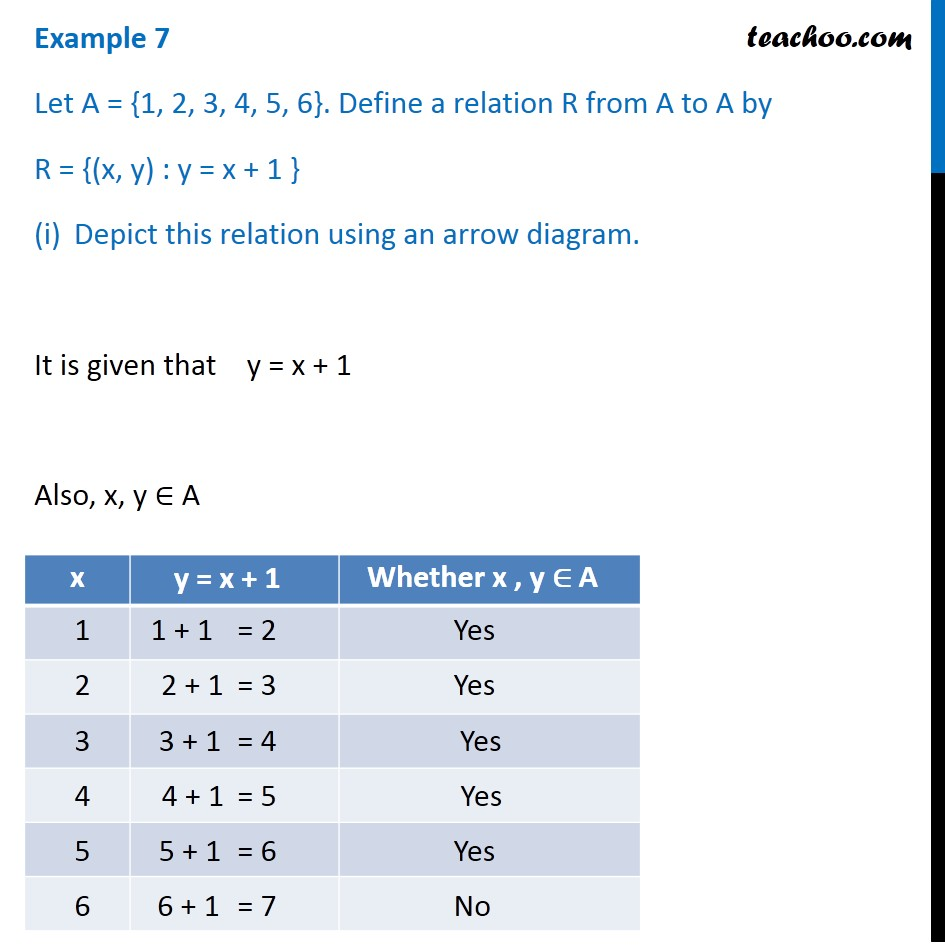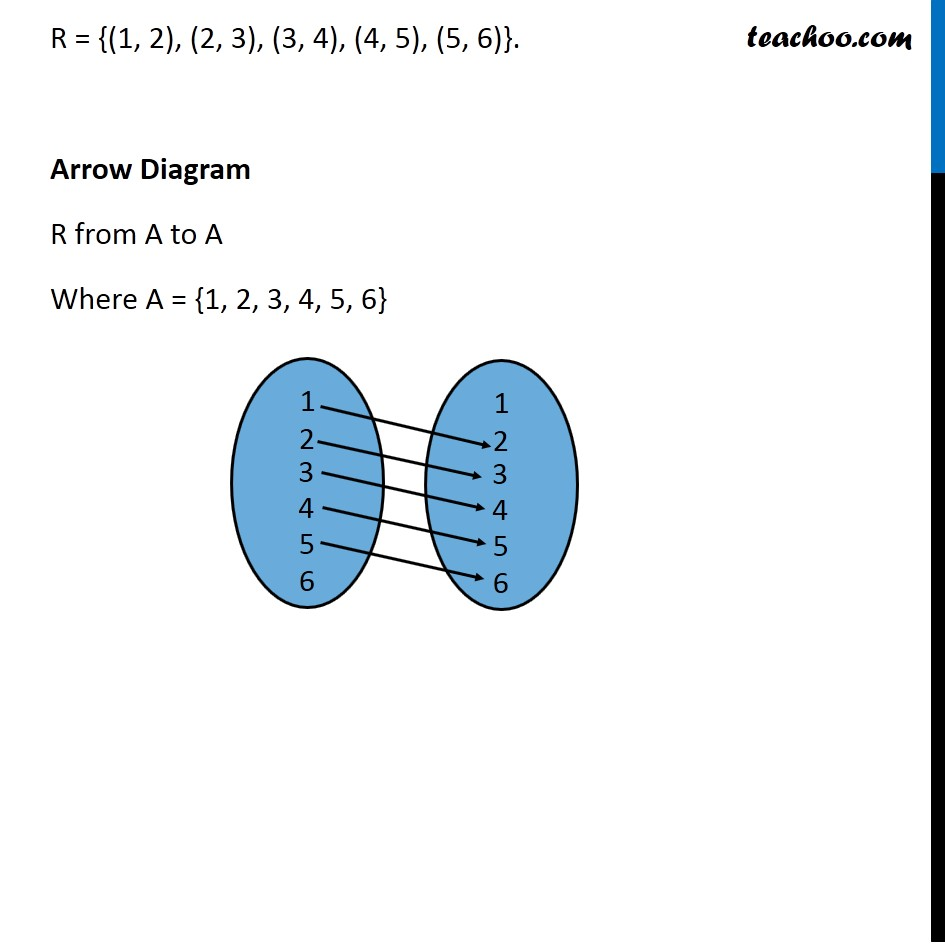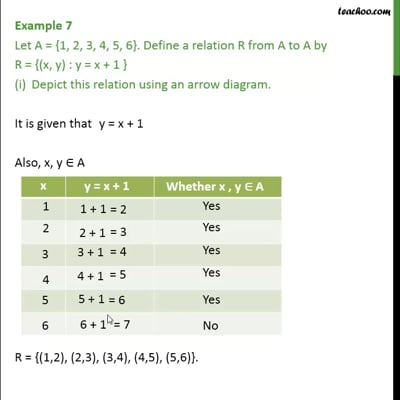This video is only available for Teachoo black users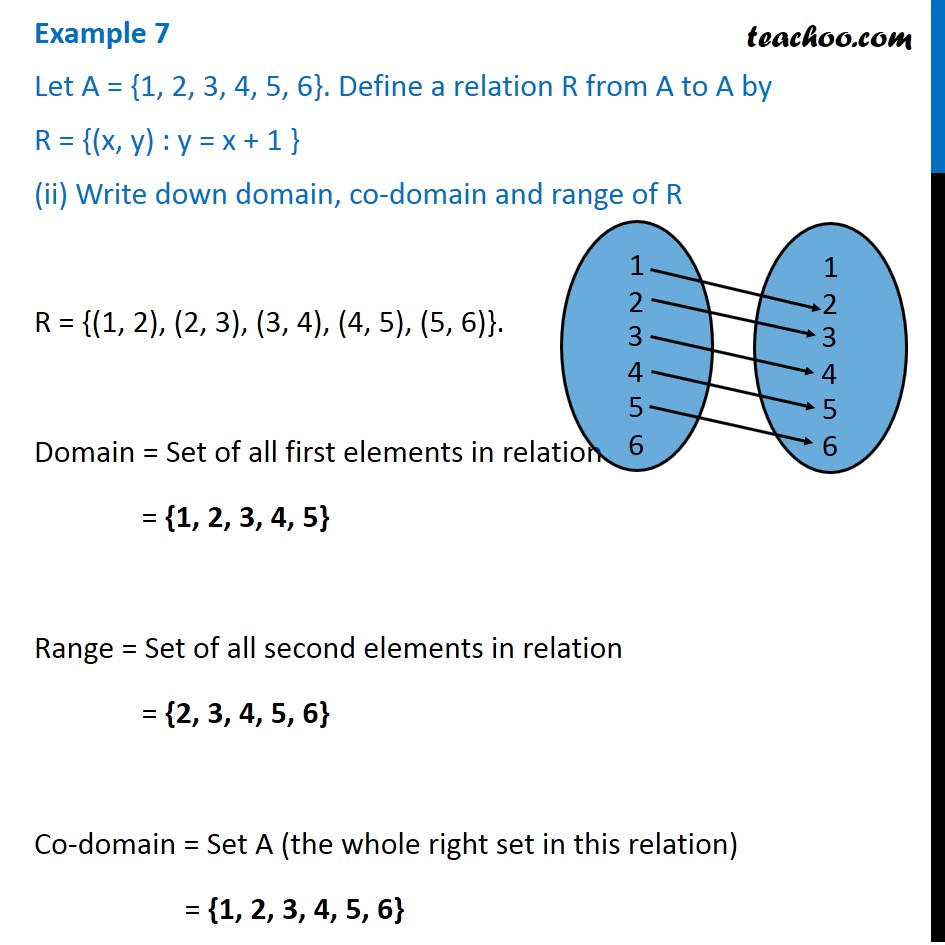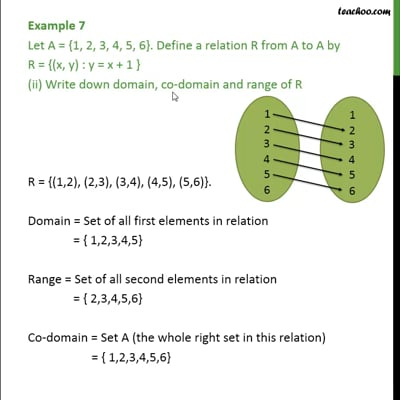This video is only available for Teachoo black users

Solve all your doubts with Teachoo Black (new monthly pack available now!)

### Transcript

Example 7 Let A = {1, 2, 3, 4, 5, 6}. Define a relation R from A to A by R = {(x, y) : y = x + 1 } Depict this relation using an arrow diagram. It is given that y = x + 1 Also, x, y ∈ A R = {(1, 2), (2, 3), (3, 4), (4, 5), (5, 6)}. Arrow Diagram R from A to A Where A = {1, 2, 3, 4, 5, 6} Example 7 Let A = {1, 2, 3, 4, 5, 6}. Define a relation R from A to A by R = {(x, y) : y = x + 1 } (ii) Write down domain, co-domain and range of R R = {(1, 2), (2, 3), (3, 4), (4, 5), (5, 6)}. Domain = Set of all first elements in relation = {1, 2, 3, 4, 5} Range = Set of all second elements in relation = {2, 3, 4, 5, 6} Co-domain = Set A (the whole right set in this relation) = {1, 2, 3, 4, 5, 6}